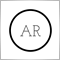Clarification on "Logical OR"38

if both conditions are true in a logical OR statement, what will be the outcome?

Eg -  if (A=1) then C=1

if (B=1) then C=1

so, accordingly,

if (A=1) || (B=0) then C should be 1

if (A=0) || (B=1) then C should be 1

if (A=1) || (B=1) then what will be the C? is it still C=1 when both conditions are true?

pls help to clarify.10580

if both conditions are true in a logical OR statement, what will be the outcome?
Eg -  if (A=1) then C=1
if (B=1) then C=1
so, accordingly,
if (A=1) || (B=0) then C should be 1
if (A=0) || (B=1) then C should be 1
if (A=1) || (B=1) then what will be the C? is it still C=1 when both conditions are true?
pls help to clarify.

OR (logical disjunction) is only looking for one of the other condition is met.

Moreover, if there are 2 conditions that are met,
then the operation is enough on the first condition: A==1,
- and B==1 will be ignored
(the process does not need to read B==1 because condition A==1 is met first.)

if(A==1||B==1) C=1; else C=0;

or

C=(A==1||B==1)? 1:0;139

According to logical OR statement

If A is FALSE  OR  B is FALSE, then C will be FALSE

If A is FALSE   OR  B is  TRUE, then C will be TRUE

If A is  TRUE   OR  B is FALSE, then C will be TRUE

If A is  TRUE  OR  B is  TRUE, then C will be TRUE

So, If (A==1 || B==1) then C=1 else C=0

if(A==1 || B==1)
C=1;
else
C=0;26723

if (A=1) then C=1

if (B=1) then C=1

Yohana Parmi #:
C=(A==1||B==1)? 1:0;

Md Atikur Rahman #:

if(A==1 || B==1)
C=1;
else
C=0;

Don't use ints when you mean bool.

C= A==1||B==1;38